# Finding similar spatial patterns

spatial
geocompr
rstats
landscape-ecology
spatial-patterns
sil
motif

TLTR:

Finding similar spatial patterns requires data for a query region and a search space. Spatial signatures are derived for the query region and many sub-areas of the search space, and distances between them are calculated. Sub-areas with the smallest distances from the query region are the most similar to it.

To reproduce the calculations in the following post, you need to download all of relevant datasets using the code below:

``````library(osfr)
dir.create("data")
osf_retrieve_node("xykzv") %>%
osf_ls_files(n_max = Inf) %>%
conflicts = "overwrite")``````

You should also attach the following packages:

``````library(sf)
library(stars)
library(tmap)``````

# Suwalski Landscape Park

Spatial pattern search allows for quantifying similarity between the query region and the search space and finally finding regions that are the most similar to the query one. Here, we were interested in finding areas of similar topography to the area of Suwalski Landscape Park. Suwalski Landscape Park is a protected area in north-eastern Poland with a post-glacial landscape consisting of young morainic hills.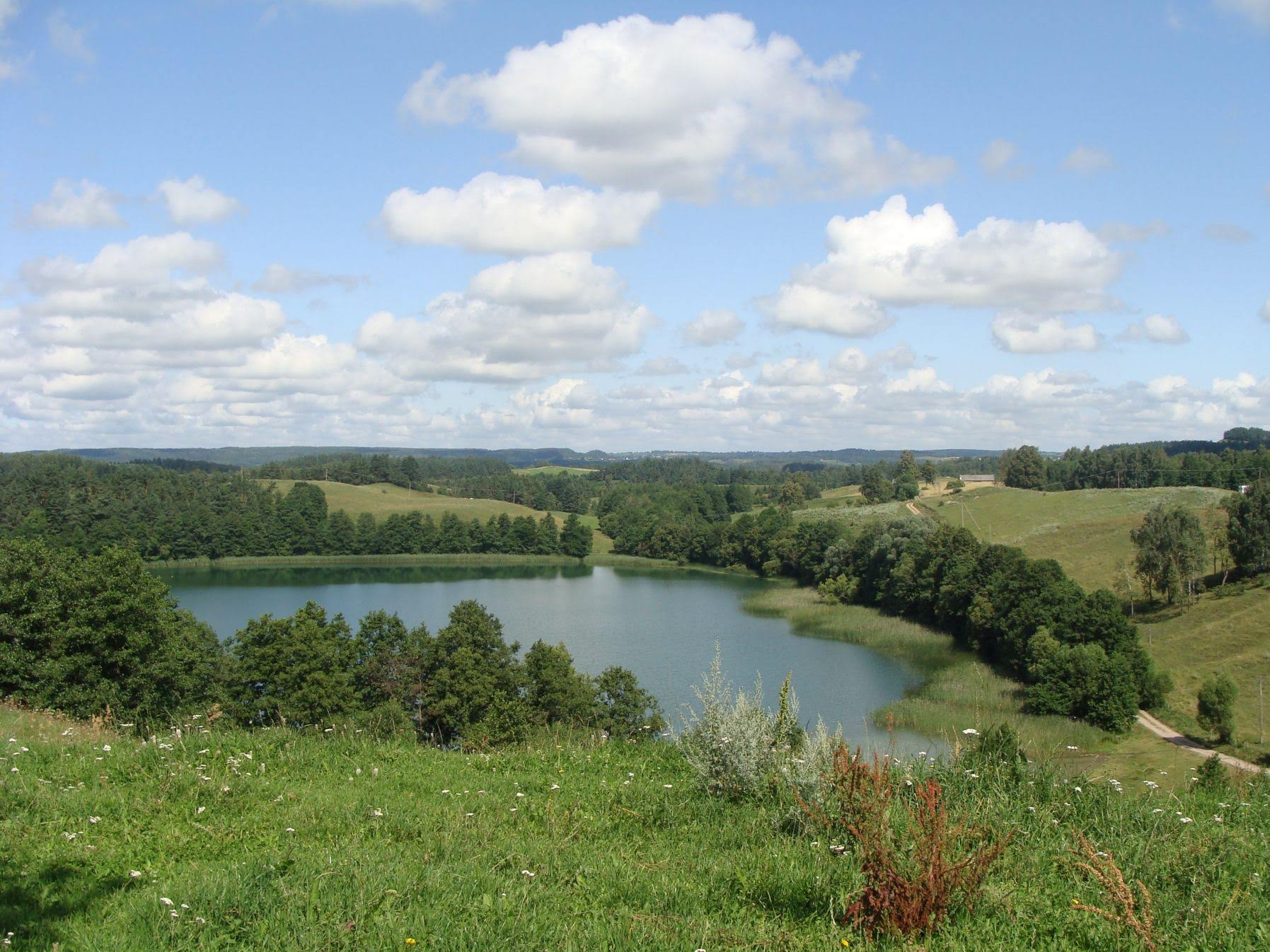One possible approach to classify the topography of a given region is to use geomorphons. Geomorphons categorize cells in this area into one of ten forms: flat, summit, ridge, shoulder, spur, slope, hollow, footslope, valley, and depression 1.

The `"data/geomorphons_pol.tif"` file contains a raster with geomorphons calculated for Poland’s area, while `"data/suw_lp.gpkg"` is a vector polygon with the Suwalski Landscape Park borders. Let’s start by reading these two files into R.

``````gm = read_stars("data/geomorphons_pol.tif")

Now, we can visualize geomorphons and the location of Suwalski Landscape Park with the tmap.

``````tm_gm = tm_shape(gm) +
tm_raster(title = "Geomorphons:") +
tm_shape(suw_lp) +
tm_symbols(col = "black", shape = 6) +
tm_layout(legend.outside = TRUE, frame = FALSE)
tm_gm``````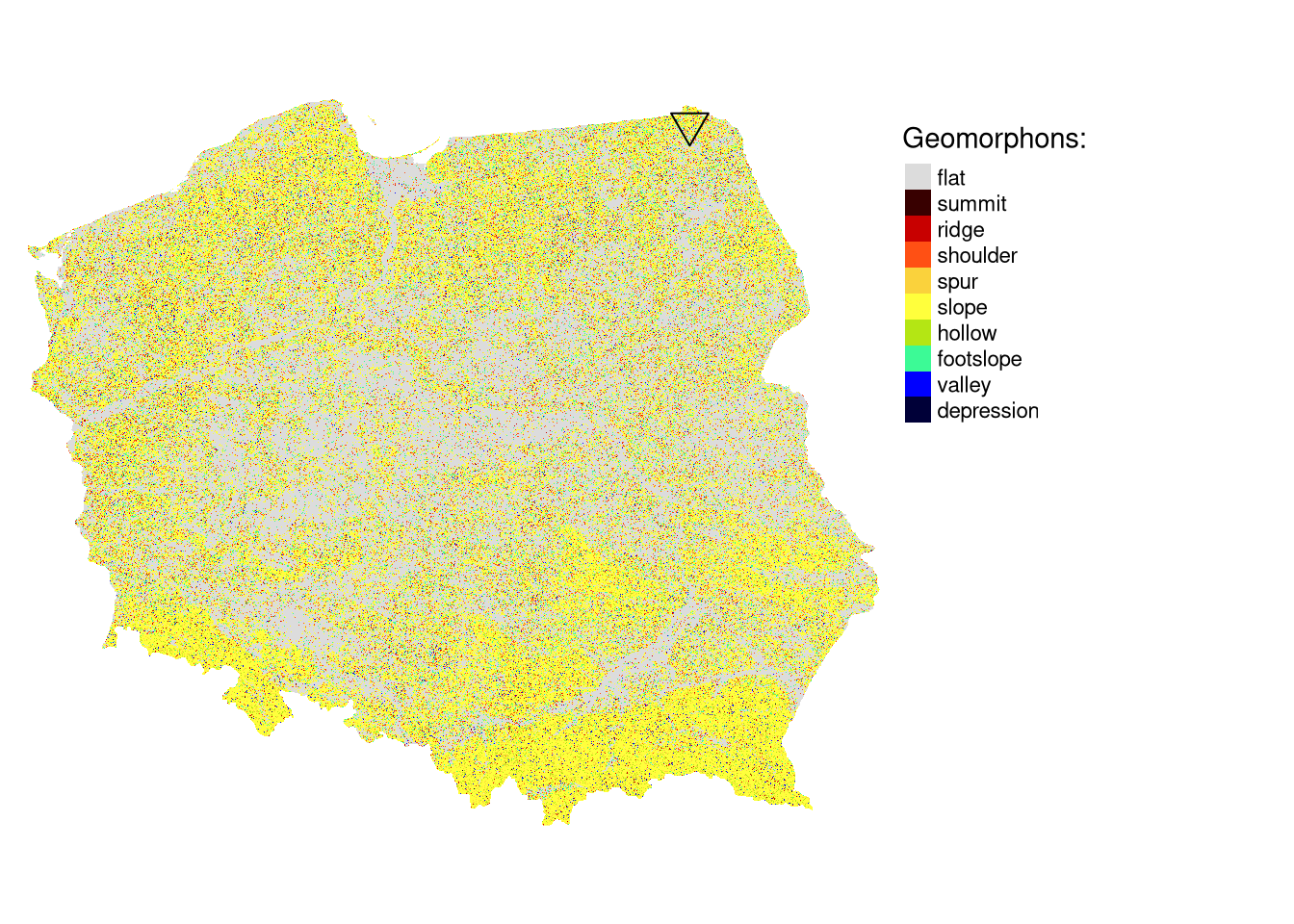# Search area

The geomorphon data for Poland is our search space. Now, we also need a second raster object with a query region. The query region is an area to which we want to find other similar areas.

There are two main ways to create a query region:

1. By cropping spatial data of a large area to the extent or borders of a query region.
2. By reading an external file. In the second case, the values in the external file should match the values in the search space.

Here, we are using the former approach by reading the Suwalski Landscape Park borders and then using it to crop the whole-country raster.

``````suw_lp = read_sf("data/suw_lp.gpkg")
gm_suw = st_crop(gm, suw_lp)``````

The query area has irregular spatial patterns represented by slopes and a limited number of flat areas.

``````tm_gm_suw = tm_shape(gm_suw) +
tm_raster() +
tm_shape(suw_lp) +
tm_borders(col = "black") +
tm_layout(legend.show = FALSE, frame = FALSE)
tm_gm_suw``````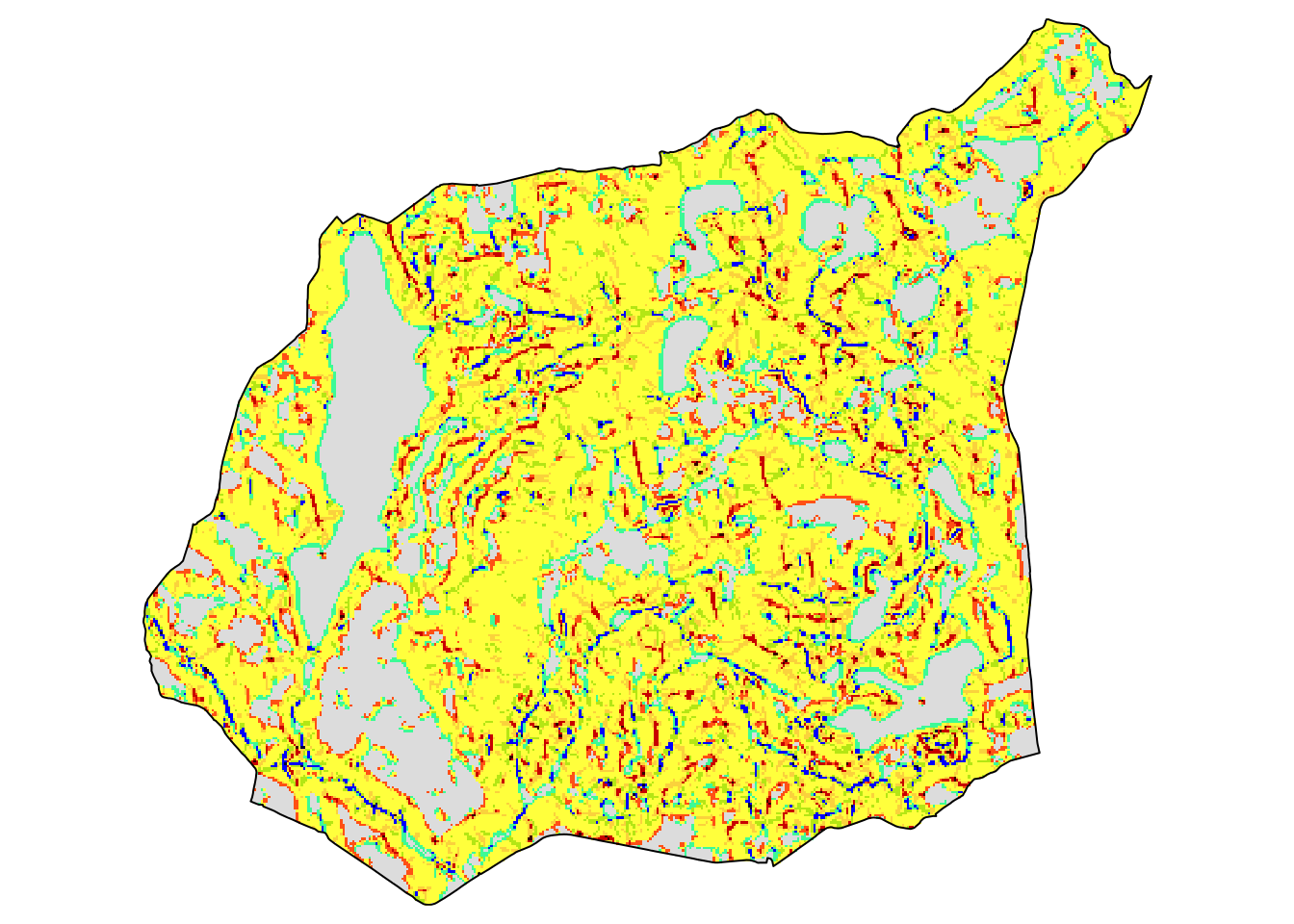# Search process

The searching process consists of:

1. Selecting a query region and a search space. In our case, the query region is `gm_sum`, while the search space is the `gm` object.
2. Dividing the search space using regular (non-overlapping) squares or using polygons.
3. Creating numerical representation (called a signature) for the query region and the search space.
4. Comparing the signature of the query region with signatures for each part of the search space using a distance measure.

# Search scale

The first important consideration is the search scale - what is the size of areas we want to find? This is not an easy question and largely depends on the research problem. The current version of motif accepts either regular (non-overlapping) squares or polygons.

# Search signature

The second consideration is the search signature. We are able to describe the above area in words, however, how to translate the spatial pattern properties to a computer and, at the same time, made them more objective? Again, it is a complex question, and largely depends on the type of input data.

In our case, we have a single categorical raster, and for this type of data, we found out that the cove signature works well. Cove stands for co-occurrence vector - it is a 1D vector where each value represents what is the share of one category is adjacent to some other cells2. More information about cove can be found in the previous blog post.

We can calculate cove for our query region using `lsp_signature()` with the `type` argument set to `"cove"`.

``````library(motif)
gm_sum_sig = lsp_signature(gm_suw, type = "cove")
gm_sum_sig``````
``````# A tibble: 1 × 3
id na_prop signature
* <int>   <dbl> <list>
1     1   0.381 <dbl [1 × 100]>``````

The output object contains a `signature` column, which stores the cove signature for our region. We can see this signature with `gm_sum_sig\$signature[]`.

# Search distance

The third consideration is a distance measure. Many distance measures have been developed for different types of data, and each of them has different properties. The motif package allows using any distance measure implemented in the philentropy package, which includes more than 40 different measures3.

# Searching

The `lsp_search()` function performs spatial pattern-based search. It expects two `stars` objects: a query region (`gm_suw`) and a search space (`gm`). Next, we need to specify the search scale (`window`), signature (`type`), and distance method (`dist_fun`).

In this example, we use a window of 100 cells by 100 cells (`window = 100`). This means that our search scale will be 2500 meters (100 cells x data resolution) - resulting in dividing the search space into about 70,000 regular rectangles of 2500 by 2500 meters. We also use the `"cove"` signature and the `"jensen-shannon"` distance here.

``````gm_search = lsp_search(gm_suw, gm,
window = 100,
type = "cove",
dist_fun = "jensen-shannon")``````

The above calculation could take several minutes on a modern computer.

# Search results

By default, the output of the search is a `stars` object with three attributes:

1. `id` - an id of each window,
2. `na_prop` - share between 0 and 1 of NA cells for each window in the search space,
3. `dist` - derived distance between the query region and each window in the search space.
``gm_search``
``````stars object with 2 dimensions and 3 attributes
attribute(s):
Min.      1st Qu.       Median         Mean      3rd Qu.
id       1.000000000 1.764075e+04 3.528050e+04 3.528050e+04 5.292025e+04
na_prop  0.000000000 0.000000e+00 0.000000e+00 3.111193e-03 0.000000e+00
dist     0.001737701 4.483980e-02 1.051218e-01 1.461936e-01 2.080158e-01
Max.  NA's
id       7.056000e+04     0
na_prop  4.997000e-01 20485
dist     6.451746e-01 20485
dimension(s):
from  to  offset delta                       refsys point values x/y
x    1 288 4595300  2500 +proj=laea +lat_0=52 +lon...    NA   NULL [x]
y    1 245 3556600 -2500 +proj=laea +lat_0=52 +lon...    NA   NULL [y]``````

We can visualize the result in the same fashion as a regular `stars` object (see the final map at the end of the post):

``````tm_search2 = tm_shape(gm_search) +
tm_raster("dist",
style = "log10",
palette = "BrBG",
title = "Distance (JSD):",
legend.is.portrait = FALSE)``````

A search result can also be easily converted into an `sf` object with `st_as_sf()`. This allows for straightforward analysis and subsetting of the search results.

``gm_search_sf = st_as_sf(gm_search)``

# The most similar areas

Spatial pattern-based search is similar to a search using internet search engines - we do not care about the most dissimilar areas. We just want to locate the ones most similar to the query region. Therefore, we should select only areas with the smallest distance values - this means that they are the most similar to the query region.

We can achieve it, for example, using the `slide_min()` function. The code below selects nine areas with the smallest distance from the query region.

``````library(dplyr)
gm_search_sel = slice_min(gm_search_sf, dist, n = 9)``````

If we want to look closer at the result, then we can extract each of the above regions with the `lsp_add_examples()` function. It adds a `region` column with a `stars` object to each row.

``gm_search_ex = lsp_add_examples(x = gm_search_sel, y = gm)``

It allows us to visualize any of the most similar areas.

``````tm_shape(gm_search_ex\$region[]) +
tm_raster() +
tm_layout(legend.show = FALSE)``````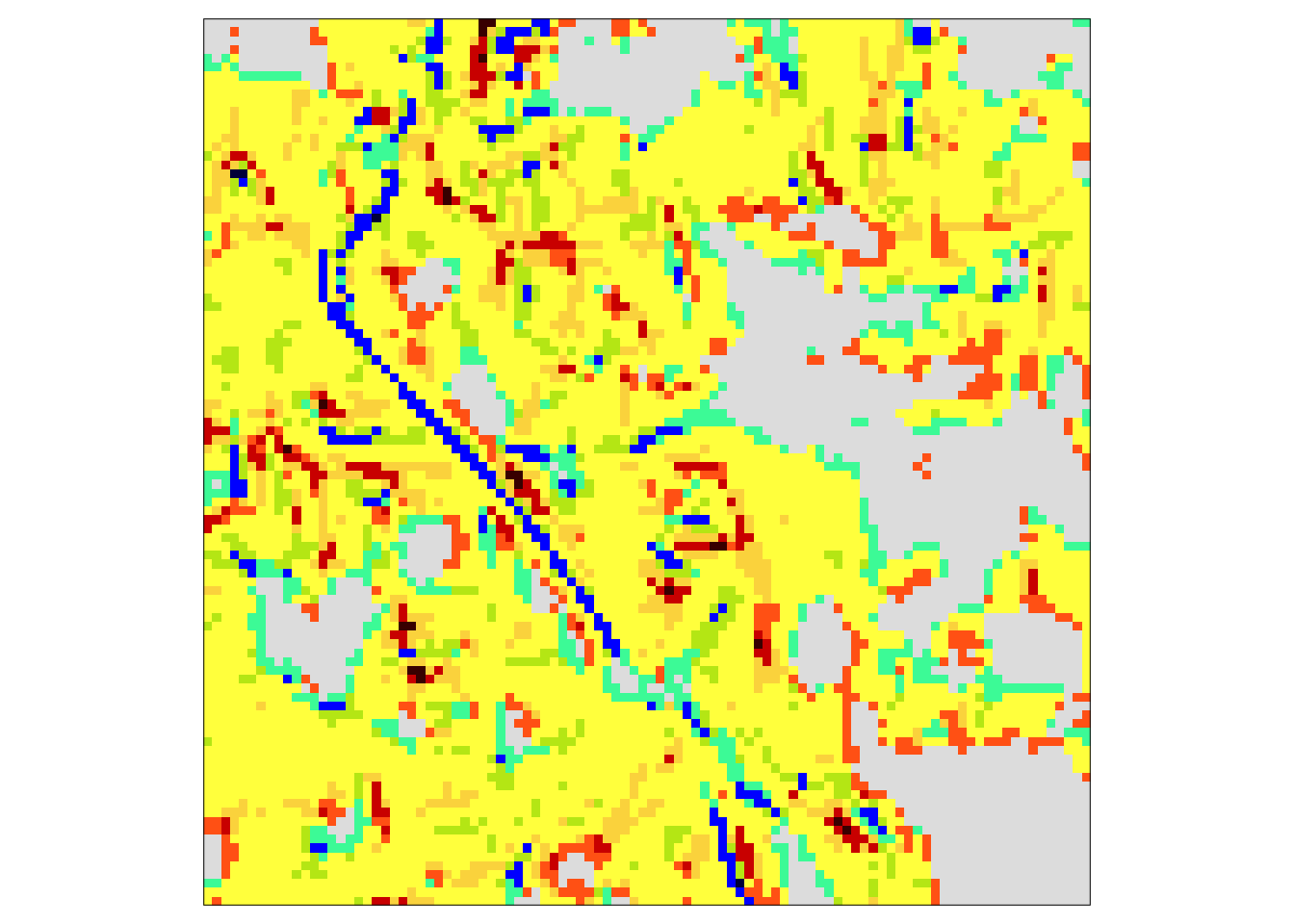This approach can also be extended to plot all nine of the most similar areas. We just need to create a visualization function (`create_map()`) and use it iteratively on each region in `gm_search_ex`. The output of this process, `map_list`, is a list of `tmap`s that can be plotted with `tmap_arrange()`:

``````library(purrr)
create_map = function(x, y){
tm_shape(x) +
tm_raster() +
tm_layout(legend.show = FALSE,
title = y)
}
map_list = map2(gm_search_ex\$region, gm_search_ex\$id, create_map)
tmap_arrange(map_list)``````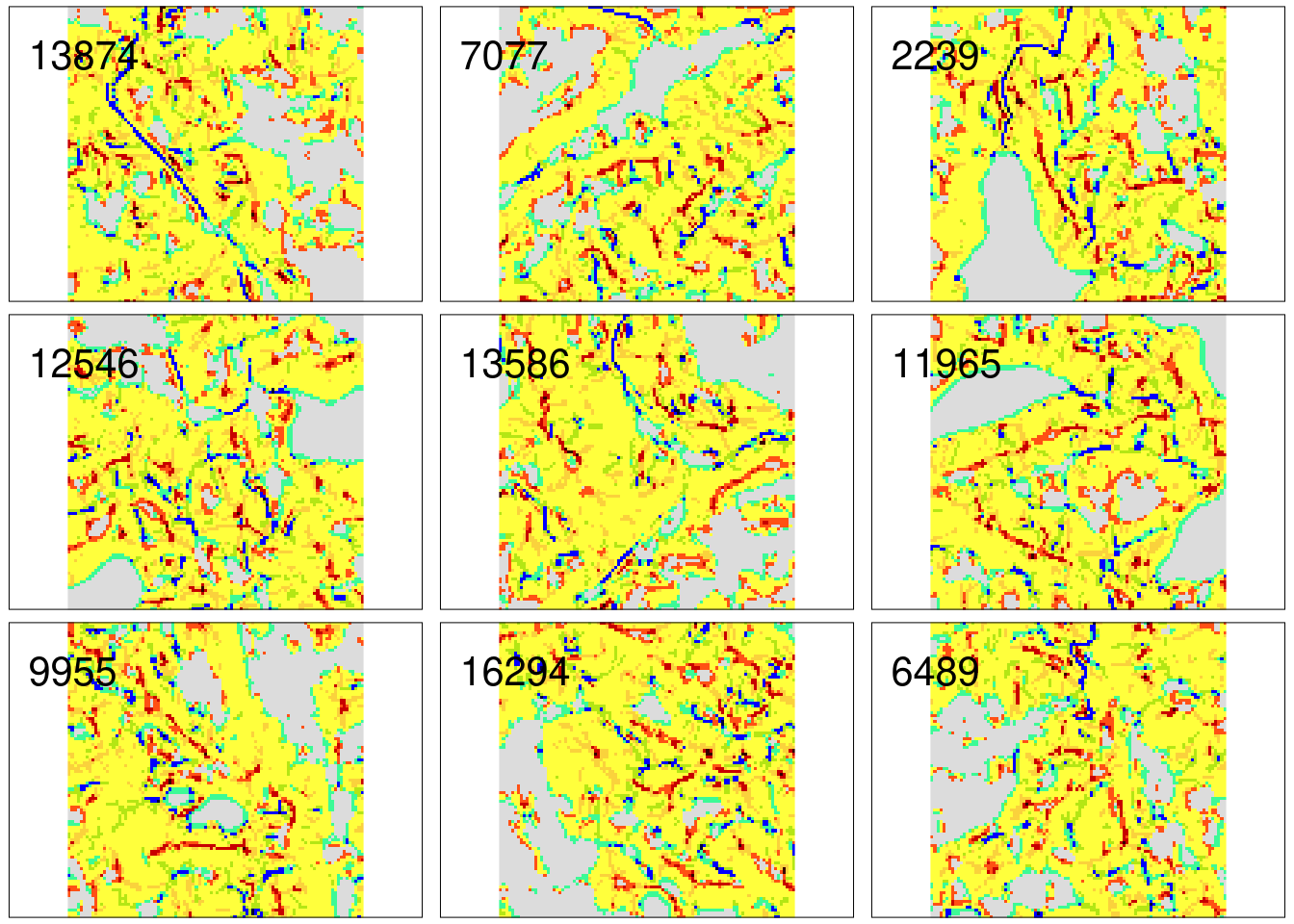Nine examples of the areas with the most similar patterns of geomorphons comparing to the Suwalski Landscape Park are presented above. They are also similar to each other, suggesting a high-quality result.

The final map consists of two parts: (a) a distance raster and (b) symbols representing the nine most similar areas.

``````tm_search2 +
tm_shape(gm_search_sel) +
tm_symbols(shape = 2, col = "black") +
tm_text("id", auto.placement = TRUE)``````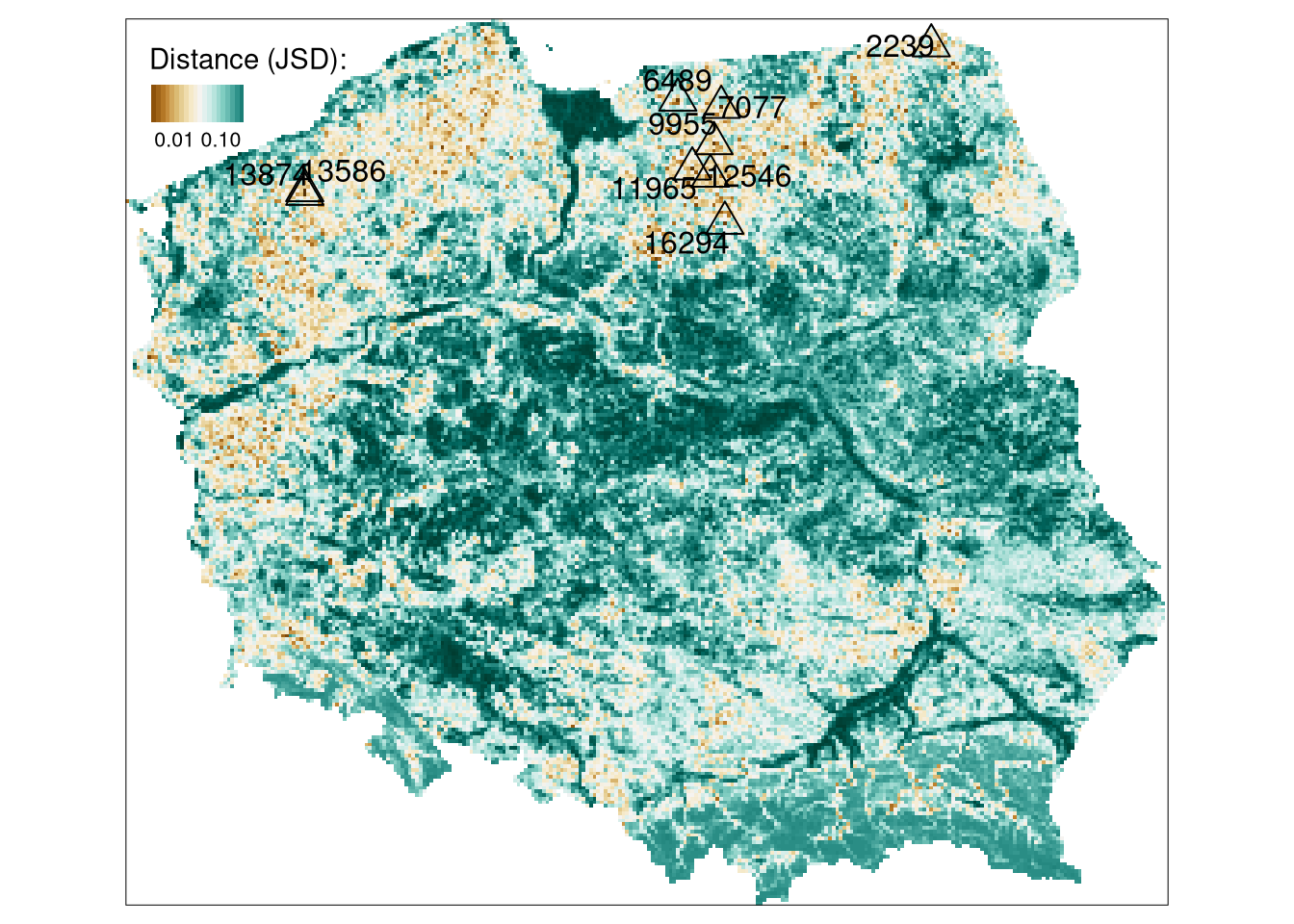The brown color on the above map represents areas with the most similar patterns of geomorphons to the Suwalski Landscape Park. The majority of similar areas are located in northern Poland and forms a belt with homogeneous topography.

# Summary

The pattern-based search allows for finding areas with similar spatial patterns. The above example shows the search based on a single variable raster data (geomorphons), but by using a different spatial signature, it can be performed on rasters with two or more variables. Additionally, search space can be not only divided into regular areas, but also in irregular ones - see an example. R code for the pattern-based search can be found here.

## Footnotes

1. Learn more about geomorphons by reading the dedicated paper or its preprint. You can also calculate geomorphons for your own data using a GRASS GIS module r.geomorphon.↩︎

2. In other words, it is a vector containing a normalized form of the co-occurrence matrix.↩︎

3. You can check all of them using `philentropy::getDistMethods()`.↩︎

## Citation

BibTeX citation:
``````@online{nowosad2021,